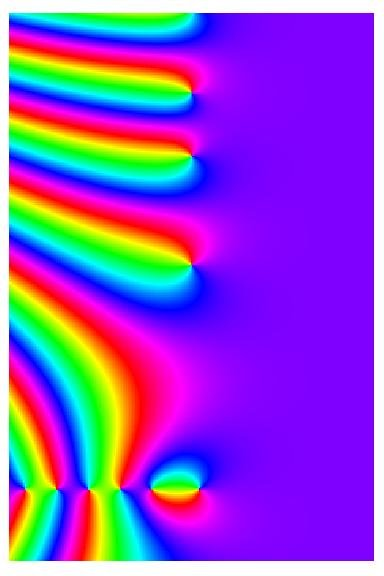How Cheenta works to ensure student success?
Explore the Back-Story

# An Introduction to Modular Forms: Research SeminarJoin this Research Seminar on "An Introduction to Modular Forms" by Subhadip Banerjee.

17th July, 2022

5 PM IST

Exclusively for College Students.

## Abstract

The theory of modular form is a central, dynamic and open topic in mathematics. Mostly being as a conjunction point of algebra and number theory, the theory of modular form is in close relation with many other important topics in mathematics such as linear algebra, complex analysis, functional analysis, topology, measure theory to name a few.

The objective of this seminar is to venture out a very minimal portion of the whole theory of modular forms.

BS and MS (dual degree programme) in Mathematics from Indian Institute of Science Education and Research (IISER), Bhopal.

Area of interests: Algebra, Number Theory, Algebraic Number Theory and Analytic Number Theory.

Join this Research Seminar on "An Introduction to Modular Forms" by Subhadip Banerjee.

17th July, 2022

5 PM IST

Exclusively for College Students.

## Abstract

The theory of modular form is a central, dynamic and open topic in mathematics. Mostly being as a conjunction point of algebra and number theory, the theory of modular form is in close relation with many other important topics in mathematics such as linear algebra, complex analysis, functional analysis, topology, measure theory to name a few.

The objective of this seminar is to venture out a very minimal portion of the whole theory of modular forms.

BS and MS (dual degree programme) in Mathematics from Indian Institute of Science Education and Research (IISER), Bhopal.

Area of interests: Algebra, Number Theory, Algebraic Number Theory and Analytic Number Theory.

This site uses Akismet to reduce spam. Learn how your comment data is processed.

### Knowledge Partner